# maths project.pptx

May. 27, 2023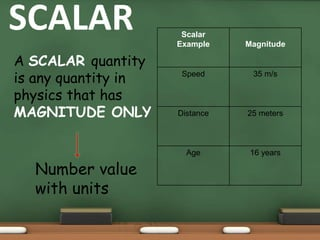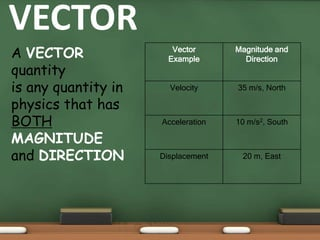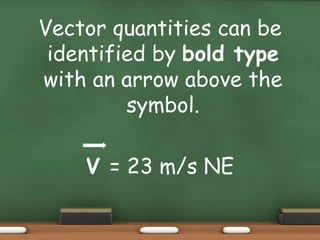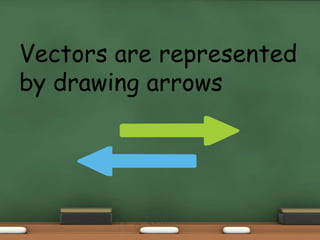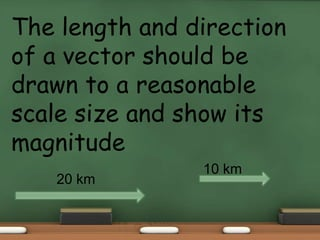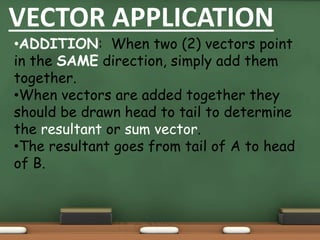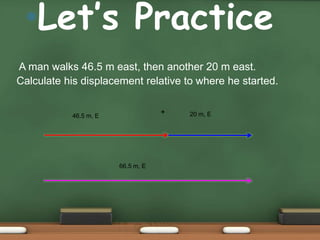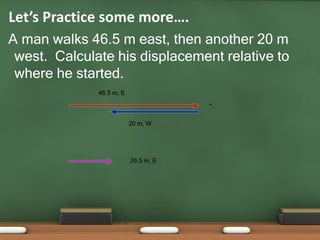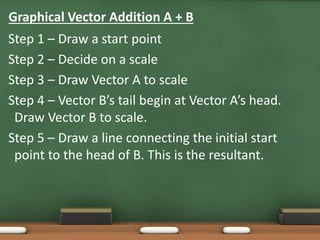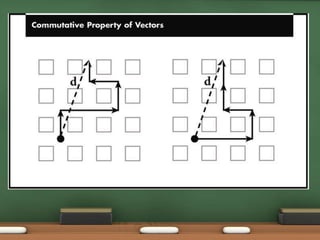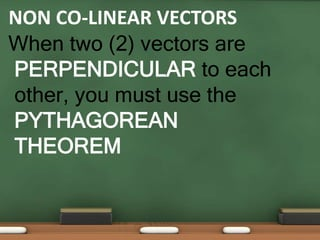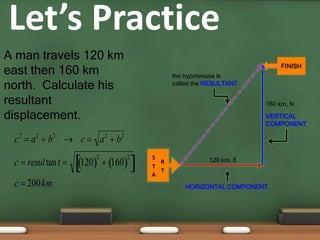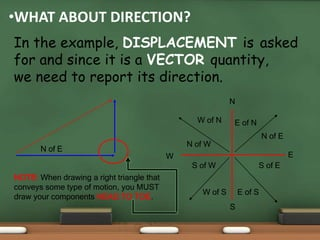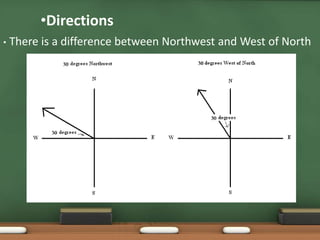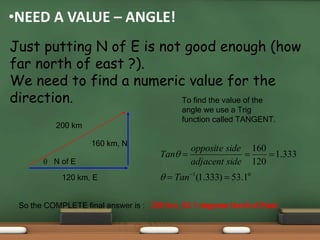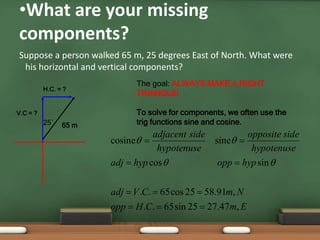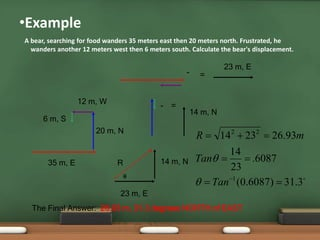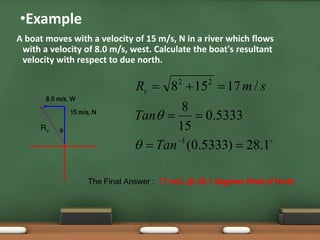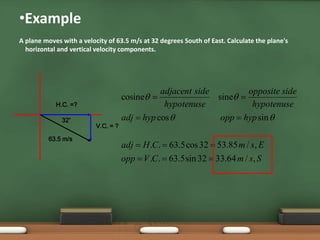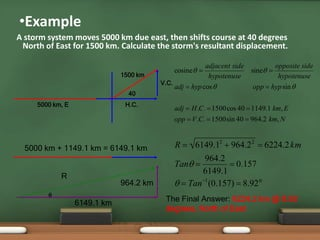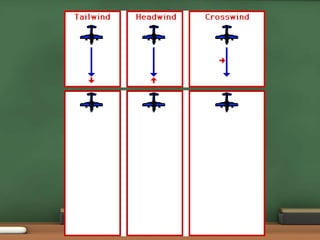1 of 24

### maths project.pptx

• 2. SCALAR A SCALAR quantity is any quantity in physics that has MAGNITUDE ONLY Number value with units Scalar Example Magnitude Speed 35 m/s Distance 25 meters Age 16 years
• 3. VECTOR A VECTOR quantity is any quantity in physics that has BOTH MAGNITUDE and DIRECTION Vector Example Magnitude and Direction Velocity 35 m/s, North Acceleration 10 m/s2, South Displacement 20 m, East
• 4. Vector quantities can be identified by bold type with an arrow above the symbol. V = 23 m/s NE
• 5. Vectors are represented by drawing arrows
• 6. The length and direction of a vector should be drawn to a reasonable scale size and show its magnitude 20 km 10 km
• 7. VECTOR APPLICATION •ADDITION: When two (2) vectors point in the SAME direction, simply add them together. •When vectors are added together they should be drawn head to tail to determine the resultant or sum vector. •The resultant goes from tail of A to head of B.
• 8. A man walks 46.5 m east, then another 20 m east. Calculate his displacement relative to where he started. •Let’s Practice 66.5 m, E 46.5 m, E + 20 m, E
• 9. •VECTOR APPLICATION SUBTRACTION: When two (2) vectors point in the OPPOSITE direction, simply subtract them.
• 10. Let’s Practice some more…. A man walks 46.5 m east, then another 20 m west. Calculate his displacement relative to where he started. 26.5 m, E 46.5 m, E - 20 m, W
• 11. Graphical Method Aligning vectors head to tail and then drawing the resultant from the tail of the first to the head of the last.
• 12. Graphical Vector Addition A + B Step 1 – Draw a start point Step 2 – Decide on a scale Step 3 – Draw Vector A to scale Step 4 – Vector B’s tail begin at Vector A’s head. Draw Vector B to scale. Step 5 – Draw a line connecting the initial start point to the head of B. This is the resultant.
• 14. NON CO-LINEAR VECTORS When two (2) vectors are PERPENDICULAR to each other, you must use the PYTHAGOREAN THEOREM
• 15. Let’s Practice A man travels 120 km east then 160 km north. Calculate his resultant displacement. c2  a2  b2  c  a2  b2 c  resultant  120   2  160   2   c  200km VERTICAL COMPONENT FINISH 120 km, E 160 km, N the hypotenuse is called the RESULTANT HORIZONTAL COMPONENT S T A R T
• 16. •WHAT ABOUT DIRECTION? In the example, DISPLACEMENT is asked for and since it is a VECTOR quantity, we need to report its direction. N S E W N of E E of N S of W W of S N of W W of N S of E E of S NOTE: When drawing a right triangle that conveys some type of motion, you MUST draw your components HEAD TO TOE. N of E
• 17. •Directions • There is a difference between Northwest and West of North
• 18. •NEED A VALUE – ANGLE! Just putting N of E is not good enough (how far north of east ?). We need to find a numeric value for the direction. 160 km, N 120 km, E To find the value of the angle we use a Trig function called TANGENT.  Tan  opposite side adjacent side  160 120 1.333   Tan1 (1.333)  53.1o N of E  200 km So the COMPLETE final answer is : 200 km, 53.1 degrees North of East
• 19. •What are your missing components? Suppose a person walked 65 m, 25 degrees East of North. What were his horizontal and vertical components? E m C H opp N m C V adj hyp opp hyp adj hypotenuse side opposite hypotenuse side adjacent , 47 . 27 25 sin 65 . . , 91 . 58 25 cos 65 . . sin cos sine cosine               65 m 25˚ H.C. = ? V.C = ? The goal: ALWAYS MAKE A RIGHT TRIANGLE! To solve for components, we often use the trig functions sine and cosine.
• 20. •Example A bear, searching for food wanders 35 meters east then 20 meters north. Frustrated, he wanders another 12 meters west then 6 meters south. Calculate the bear's displacement.  3 . 31 ) 6087 . 0 ( 6087 . 23 14 93 . 26 23 14 1 2 2         Tan Tan m R   35 m, E 20 m, N 12 m, W 6 m, S - = 23 m, E - = 14 m, N 23 m, E 14 m, N The Final Answer: 26.93 m, 31.3 degrees NORTH of EAST R 
• 21. •Example A boat moves with a velocity of 15 m/s, N in a river which flows with a velocity of 8.0 m/s, west. Calculate the boat's resultant velocity with respect to due north.  1 . 28 ) 5333 . 0 ( 5333 . 0 15 8 / 17 15 8 1 2 2         Tan Tan s m Rv   15 m/s, N 8.0 m/s, W Rv  The Final Answer : 17 m/s, @ 28.1 degrees West of North
• 22. •Example A plane moves with a velocity of 63.5 m/s at 32 degrees South of East. Calculate the plane's horizontal and vertical velocity components. S s m C V opp E s m C H adj hyp opp hyp adj hypotenuse side opposite hypotenuse side adjacent , / 64 . 33 32 sin 5 . 63 . . , / 85 . 53 32 cos 5 . 63 . . sin cos sine cosine               63.5 m/s 32˚ H.C. =? V.C. = ?
• 23. •Example A storm system moves 5000 km due east, then shifts course at 40 degrees North of East for 1500 km. Calculate the storm's resultant displacement. N km C V opp E km C H adj hyp opp hyp adj hypotenuse side opposite hypotenuse side adjacent , 2 . 964 40 sin 1500 . . , 1 . 1149 40 cos 1500 . . sin cos sine cosine                R  6149.12  964.22  6224.2km Tan  964.2 6149.1  0.157   Tan1 (0.157)  8.92o 5000 km, E 40 1500 km H.C. V.C. 5000 km + 1149.1 km = 6149.1 km 6149.1 km 964.2 km R  The Final Answer: 6224.2 km @ 8.92 degrees, North of East